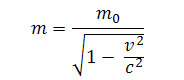Modern Physics

# Relativistic MassEquation for calculating relativistic mass

When we apply a force of magnitude F to a body, we make it acquire speed, or rather, we can increase its speed indefinitely. Now, if a body reached the speed of light in a vacuum, the force would no longer be able to accelerate it, because the speed limit was reached, taking into account the Theory of Relativity.

In this case, we could say that the inertia of this body would be finite. Therefore, every time we increase the speed of a body, we also increase its inertia. If we continue to increase its speed, tending to the speed of light, its inertia will tend to become infinitely large.

We learned that the mass of a body is nothing more than the inertia of that body. In this way, 0corresponds to the mass of a certain body at rest in relation to an inertial frame of reference and m is its mass when endowed with velocity v . The masses m and m0 are related according to the above equation.

In the equation, or rather in this formula, 0 is called the rest mass and m , the relativistic mass.

How:Note that, since the speed v is negligible when compared with c , we can do:Under these conditions, m = m 0 results according to Classical Mechanics. On the other hand, as v tends to c , the root tends to zero and tends to infinity.